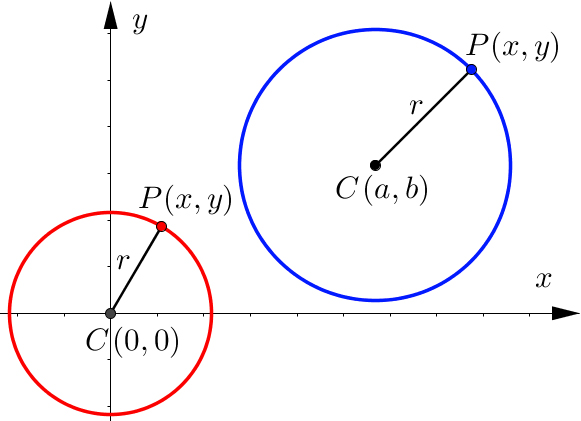# Circle

Keywords:The equation of a centred circle

${x}^{2}+{y}^{2}={r}^{2}$

The general equation of a circle

$\left(x-a{\right)}^{2}+\left(y-b{\right)}^{2}={r}^{2}$